1. /
2. CBSE
3. /
4. Class 08
5. /
6. Mathematics
7. /
8. NCERT Solutions for Class...

# NCERT Solutions for Class 8 Maths Exercise 12.2### myCBSEguide App

Download the app to get CBSE Sample Papers 2023-24, NCERT Solutions (Revised), Most Important Questions, Previous Year Question Bank, Mock Tests, and Detailed Notes.

NCERT solutions for Class 8 Maths Exponents and Powers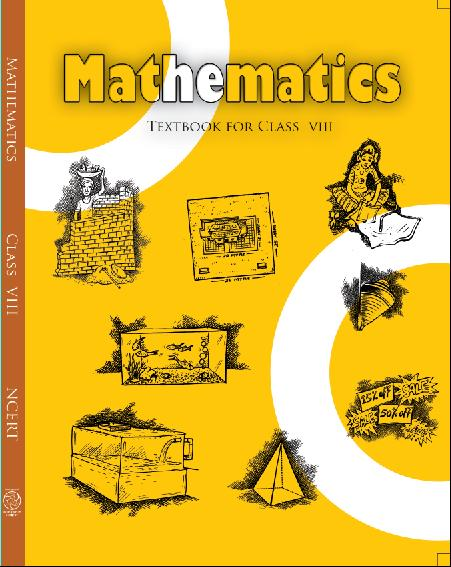## NCERT Solutions for Class 8 Maths Exponents and Powers

###### 1. Express the following numbers in standard form:

(i)   0.0000000000085

(ii)  0.00000000000942

(iii) 6020000000000000

(iv) 0.00000000837

(v)  31860000000

Ans. (i) 0.0000000000085

= 0.0000000000085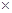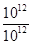=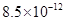(ii) 0.00000000000942

= 0.00000000000942=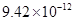(iii) 6020000000000000

= 6020000000000000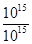=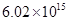(iv)  0.00000000837

= 0.00000000837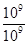=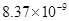(v) 31860000000 = 31860000000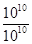=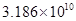NCERT Solutions for Class 8 Maths Exercise 12.2

###### 2. Express the following numbers in usual form:

(i)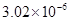(ii)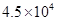(iii)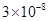(iv)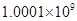(v)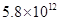(vi)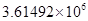Ans. (i)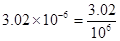= 0.00000302

(ii)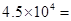4.510000 = 45000

(iii)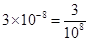= 0.00000003

(iv)= 1000100000

(v)= 5.8 x 1000000000000

= 5800000000000

(vi)= 3.614921000000

= 3614920

NCERT Solutions for Class 8 Maths Exercise 12.2

###### 3. Express the number appearing in the following statements in standard form:

(i)1 micron is equal to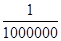m.

(ii) Charge of an electron is    0.000,000,000,000,000,000,16 coulomb.

(iii) Size of a bacteria is 0.0000005 m.

(iv) Size of a plant cell is

0.00001275 m.

(v) Thickness if a thick paper is 0.07 mm.

Ans. (i) 1 micron

=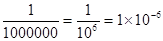m

(ii)Charge of an electron is

0.00000000000000000016 coulombs.

= 0.00000000000000000016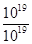=coulomb

(iii) Size of bacteria = 0.0000005

=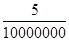=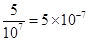m

(iv) Size of a plant cell is 0.00001275 m

= 0.00001275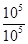=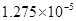m

(v) Thickness of a thick paper = 0.07 mm

=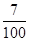mm =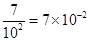mm

NCERT Solutions for Class 8 Maths Exercise 12.2

###### 4. In a stack there are 5 books each of thickness 20 mm and 5 paper sheets each of thickness 0.016 mm. What is the total thickness of the stack?

Ans. Thickness of one book = 20 mm

Thickness of 5 books = 205 = 100 mm

Thickness of one paper = 0.016 mm

Thickness of 5 papers = 0.0165

= 0.08 mm

Total thickness of a stack = 100 + 0.08

=100.08 mm

= 100.08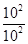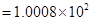mm

## NCERT Solutions for Class 8 Maths Exercise 12.2

NCERT Solutions Class 8 Mathematics PDF (Download) Free from myCBSEguide app and myCBSEguide website. Ncert solution class 8 Mathematics includes text book solutions from Class 8 Maths Book . NCERT Solutions for CBSE Class 8 Maths have total 16 chapters. 8 Maths NCERT Solutions in PDF for free Download on our website. Ncert class 8 solutions PDF and Maths ncert class 8 PDF solutions with latest modifications and as per the latest CBSE syllabus are only available in myCBSEguide.

## CBSE app for Class 8

To download NCERT Solutions for class 8 Social Science, Computer Science, Home Science,Hindi ,English, Maths Science do check myCBSEguide app or website. myCBSEguide provides sample papers with solution, test papers for chapter-wise practice, NCERT solutions, NCERT Exemplar solutions, quick revision notes for ready reference, CBSE guess papers and CBSE important question papers. Sample Paper all are made available through the best app for CBSE students and myCBSEguide website.Test Generator

Create question paper PDF and online tests with your own name & logo in minutes.myCBSEguide

Question Bank, Mock Tests, Exam Papers, NCERT Solutions, Sample Papers, Notes

### 10 thoughts on “NCERT Solutions for Class 8 Maths Exercise 12.2”

1. It is godd

2. 1 mistake write 2 answers of one question ,because people which Answer like best they write. Please do this

3. Nice app. It helps for evrything. We can ask ask any thing from the app. It is always correct.

4. I love this app it helps me very much I can understand maths very easily here

5. I love this app it helps me in my maths work I can understand maths very easily on this app

6. I love this app it helps me in my maths work I can understand maths very easily on this app

7. It is easy for learn

8. it is easy for learning , i like this app……………………….

9. I luv this app whenever i have doubt i see it n clr my doubt

10. It is superb